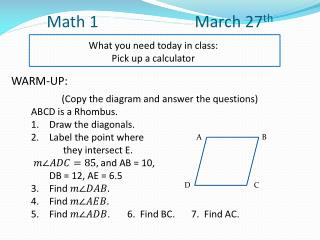DownloadDownload PresentationMath 1 March 27 th

# Math 1 March 27 th

Télécharger la présentation## Math 1 March 27 th

- - - - - - - - - - - - - - - - - - - - - - - - - - - E N D - - - - - - - - - - - - - - - - - - - - - - - - - - -
##### Presentation Transcript

1. Math 1 March 27th What you need today in class: Pick up a calculator WARM-UP: (Copy the diagram and answer the questions) ABCD is a Rhombus. Draw the diagonals. Label the point where they intersect E. and AB = 10, DB = 12, AE = 6.5 Find Find Find . 6. Find BC. 7. Find AC. A B D C

2. Check homework • 30 • 90 • 6 • 4 • 150 • 90 • 6 • 4 • 70.6 • 9 • 90 • 35.3

3. pg. 11-12 PARALLELOGRAMS CHARACTERISTICS 1 – Opposite sides are ___ 2 – Opposite sides are ___ 3 – Opposite angles are __ 4 – Consecutive angles are __ 5 – Diagonals ___ each other

4. RECTANGLE CHARACTERISTICS 1 – All angle are ____. 2 – Diagonals are ____.

5. RHOMBUS CHARACTERISTICS 1 – All sides are ____. 2 – Diagonals are ____. 3 – Diagonals bisect ____ angles.

6. SQUARE CHARACTERISTICS 1 – 2 – 3 – 4 – 5 –

7. Review for Test • Find these Quizzes • Points of Concurrency • Polygons • Notes • Pages 4 – 5 • Pages 16-17 • Pages 21 and 2-page handout

8. Review What is a point of concurrency? Match these: • Circumcenter A. Angle bisectors • Orthocenter B. Perpendicular Bisectors • Centroid C. Altitudes • Incenter D. Medians

9. Review • Which point of concurrency is equal distance from each angle? • Which point of concurrency is equal distance from each side?

10. Review

11. Review • How many sides? • Pentagon • Heptagon • Dodecagon • Decagon • Hexagon

12. Review • Sum of interior angles of a 15-gon? • Sum of exterior angles of a hexagon? • Measure of one interior angle of a 15-gon? • Measure of one exterior angle of a 14-gon? • The sum of the measures of a polygon is 1980. How many sides does the polygon have?

13. Review • If the measure of one exterior angle of a regular polygon is 45°, then how many sides does the polygon have? • If the measure of one interior angle of a regular polygon is 150°, then how many sides does the polygon have?